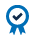##### Education Home

Market participants trade in the futures market to make a profit or hedge against losses. Each market calculates movement of price and size differently, and as such, traders need to be aware of how the market you are trading calculates profit and loss. To determine the profit and loss for each contract, you will need to be aware of the contract size, tick size, current trading price, and what you bought or sold the contract for. WTI Crude Oil futures, for example, represents the expected value of 1,000 barrels of oil. The price of a WTI futures contract is quoted in dollars per barrel. The minimum tick size is \$0.01.

## Current Value

If the current price of WTI futures is \$54, the current value of the contract is determined by multiplying the current price of a barrel of oil by the size of the contract. In this example, the current value would be \$54 x 1000 = \$54,000.

## Value of a One-Tick Move

The dollar value of a one-tick move is calculated by multiplying the tick size by the size of the contract.

The dollar value of a one-tick move in WTI is \$0.01 x 1000 = \$10

## Calculation Example

Calculating profit and loss on a trade is done by multiplying the dollar value of a one-tick move by the number of ticks the futures contract has moved since you purchased the contract. This calculation gives you profit or loss per contact, then you need to multiply this number by the number of contracts you own to get the total profit or loss for your position.

The price of WTI is now \$54.

The profit-per-contract for the trader is \$54.00-53.60 = \$0.40

Therefore, the contract has moved \$0.40 divided by \$0.01 = 40 ticks

The total move in dollars is 40 ticks x \$10 per tick = \$400

The total profit would be \$400 x the number of contracts the trader owns

Losses are calculated in the same manner as gains.

## The Value of Your Position

The size of the contract can have a considerable multiplying effect on the profit and loss of a specific futures contract. Before entering a position in the futures market, it is critical that you understand how any price fluctuation or market volatility affects the value of your open trading position. Consider the average price move for the contract and the corresponding tick value to understand the size of typical moves and its value.

For example, the 14-day average true range is 15 for the ES and 0.32 for Silver futures (SI) .

The calculation is as follows:

The value of a typical daily move in dollars for the ES contract = 7.5 points x \$50 per point = \$375

Compared to the ES contract, the SI contract is a larger contract with larger moves.

The average true range or ATR for the SI contract  \$0.16 = 160 ticks

The value of a typical daily move in dollars is 160 ticks x \$5 per tick = \$800

This example illustrates that on average the ES contract moves less than half the dollar value of the SI contract.

Some average moves in the larger valued futures contracts can be sizable, and traders should plan their risk and reward accordingly.

#### ACCREDITED COURSEDid you know that CME Institute classes can fulfill CFA and GARP continuing education requirements? Every CME Institute course can be self-reported in your CFA online CE tracker and select classes can be used for GARP credits. See which of our classes qualify for GARP credits here.